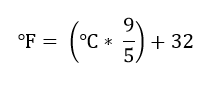# Basic Java programming exercises

 Java Tutorial Java Exercises Data Structures

## Java program to convert temperature from degree Celsius to Fahrenheit

Write a Java program to input temperature in Centigrade and convert to Fahrenheit. How to convert temperature from degree centigrade to degree Fahrenheit in Java programming. Logic to convert temperature from Celsius to Fahrenheit in Java.

##### Required knowledge

Arithmetic operators, Variables and expressions, Data types, Basic input/output

##### Temperature conversion formula

Temperature conversion formula from degree Celsius to Fahrenheit is given by -##### Logic to convert temperature from Celsius to Fahrenheit

The logic of temperature conversion exists in converting mathematical formula to C expression. You just need to convert mathematical formula of temperature in C language. Rest is simple input/output.

Below is the step by step descriptive logic to convert temperature from degree Celsius to Fahrenheit.

1. Input temperature in Celsius from user. Store it in some variable say celsius.

2. Apply formula to convert the temperature to Fahrenheit i.e. fahrenheit = (celsius * 9 / 5) + 32.

3. Print the value of fahrenheit.

```Read more - Program to convert Fahrenheit to Celsius
```

##### Program to convert temperature from Celsius to Fahrenheit
```/**
* Java program to convert temperature from degree celsius to fahrenheit
*/

import java.util.Scanner;

class Test
{
public static void main(String args[])
{
double celsius, fahrenheit;
Scanner op=new Scanner(System.in);
/* Input temperature in celsius */
System.out.print("Enter temperature in Celsius: ");
celsius=op.nextDouble();

/* celsius to fahrenheit conversion formula */
fahrenheit = (celsius * 9 / 5) + 32;

System.out.print(celsius+" Celsius = "+fahrenheit+" Fahrenheit");
}
}
```

##### Output:

```Enter temperature in Celsius: 31
31.0 Celsius = 87.8 Fahrenheit
```